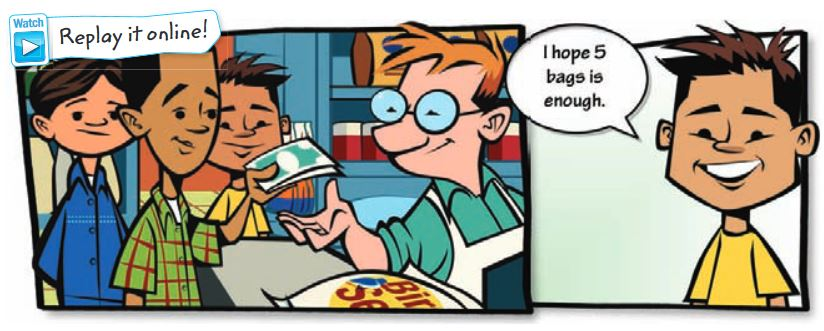Homework Explained - Math Practice 101Dear guest, you are not a registered member. As a guest, you only have read-only access to our books, tests and other practice materials.

As a registered member you can:

Registration is free and doesn't require any type of payment information. Click here to Register.
Go to page:
Chapter 4: Multiply and Divide Fractions;Lesson 1: Estimate Products of Fractions

Estimate each product. Use a bar diagram if needed.

•  $$\large \frac{1}{4}\times21\approx$$
•  $$\large \frac{5}{7}\text{ of }22\approx$$
•  $$\large \frac{5}{7}\times\frac{1}{9}\approx$$
•  $$\large 4\frac{1}{3}\times2\frac{3}{4}\approx$$
• Question 5

Cyrus is inviting 11 friends over for pizza. He would like to have enough pizza so each friend can have $$\frac{1}{4}$$ of a pizza. About how many pizzas should he order?

• Question 6

Hakeem's front porch measures $$9\frac{3}{4}$$ feet by 4 feet. Estimate the area of his front porch.

Use Math Tools Refer to the graphic novel frame for Exercises a–b.• Question 7

a. If each bag holds $$3\frac{3}{4}$$ pounds, estimate how many pounds of birdseed Elisa, Luis, and Dwayne purchased.

b. Suppose each bag costs $14.99. Estimate the total cost of 5 bags. •  about$